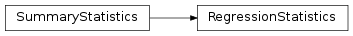# mvpa2.clfs.base.RegressionStatistics¶class `mvpa2.clfs.base.``RegressionStatistics`(**kwargs)

Class to contain information and display on regression results.

Attributes

 `error` `sets` `stats` `summaries` Return a list of separate summaries per each stored set

Methods

 `add`(targets, predictions[, estimates]) Add new results to the set of known results `as_string`([short, header, summary, description]) ‘Pretty print’ the statistics `compute`() Actually compute the confusion matrix based on all the sets `plot`([plot, plot_stats, splot]) Provide presentation of regression performance in image `reset`() Cleans summary – all data/sets are wiped out

Initialize RegressionStatistics

Parameters: targets Optional set of targets predictions Optional set of predictions

Attributes

 `error` `sets` `stats` `summaries` Return a list of separate summaries per each stored set

Methods

 `add`(targets, predictions[, estimates]) Add new results to the set of known results `as_string`([short, header, summary, description]) ‘Pretty print’ the statistics `compute`() Actually compute the confusion matrix based on all the sets `plot`([plot, plot_stats, splot]) Provide presentation of regression performance in image `reset`() Cleans summary – all data/sets are wiped out
`as_string`(short=False, header=True, summary=True, description=False)

‘Pretty print’ the statistics

`error`
`plot`(plot=True, plot_stats=True, splot=True)

Provide presentation of regression performance in image

Parameters: plot : bool Plot regular plot of values (targets/predictions) plot_stats : bool Print basic statistics in the title splot : bool Plot scatter plot (fig, im, cb) – figure, imshow, colorbar